# Volumetric Gravimetric Analysis VOLUMETRIC GRAVIMETRIC ANALYSIS Laboratory problem

• Slides: 11Volumetric & Gravimetric Analysis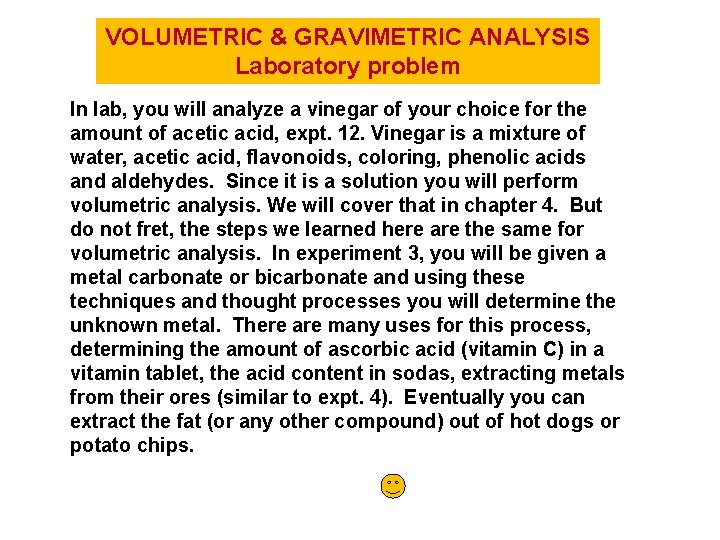VOLUMETRIC & GRAVIMETRIC ANALYSIS Laboratory problem In lab, you will analyze a vinegar of your choice for the amount of acetic acid, expt. 12. Vinegar is a mixture of water, acetic acid, flavonoids, coloring, phenolic acids and aldehydes. Since it is a solution you will perform volumetric analysis. We will cover that in chapter 4. But do not fret, the steps we learned here are the same for volumetric analysis. In experiment 3, you will be given a metal carbonate or bicarbonate and using these techniques and thought processes you will determine the unknown metal. There are many uses for this process, determining the amount of ascorbic acid (vitamin C) in a vitamin tablet, the acid content in sodas, extracting metals from their ores (similar to expt. 4). Eventually you can extract the fat (or any other compound) out of hot dogs or potato chips.GRAVIMETRIC ANALYSIS 1. Aluminum can be determined gravimetrically by reaction with a solution of 8 -hydroxyquinoline (C 9 H 7 NO). A mass of 0. 1248 g of Al(C 9 H 7 NO)3 was obtained by precipitating all of the Al 3+ from a solution prepared by dissolving 1. 8571 g of a mineral. What is the mass percent of aluminum in the mineral? mineral + C 9 H 7 NO 0. 01248 g C* 1 mol C 46 g C → Al 1 mol Al 2 mol C 27. 0 g Al 1 mol Al = 7. 293 x 10 -3 g Al % Al = mass Al mass mineral 7. 293 x 10 -3 g Al = 1. 8571 g mineral *To simplify the set up I am going to call C = Al(C 9 H 7 NO)3 0. 3928%GRAVIMETRIC ANALYSIS – Lecture problems 1) A particular coal contains 2. 8% sulfur by weight. When this coal is burned, the sulfur appears as sulfur dioxide gas. This gas then reacts with calcium oxide to form solid calcium sulfite. If the coal is burned in a power plant that used 200 kg/hour of coal, how much calcium sulfite is produced in 2 hours? Step 1: Since a balanced equation is not possible; in gravimetric analysis the first step is to draw out a plan, draw a picture to represent the physical process in the lab. ORE compound solution Step 2: Find correlations and relationships (maybe common element) Step 3: Stoichiometry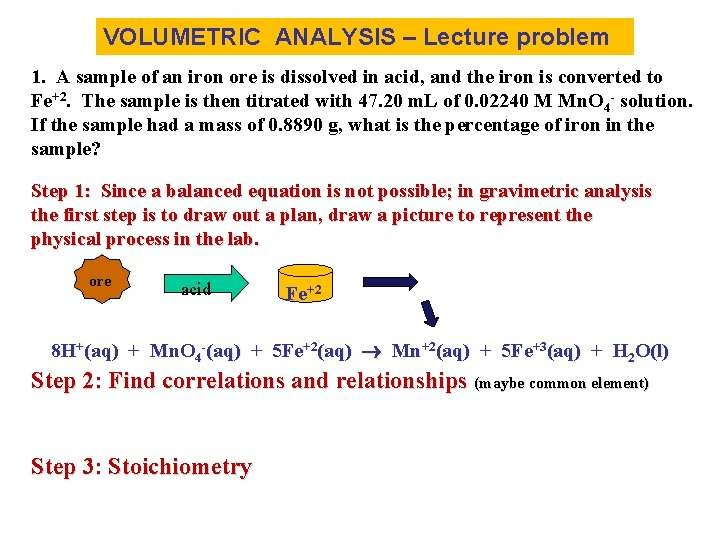VOLUMETRIC ANALYSIS – Lecture problem 1. A sample of an iron ore is dissolved in acid, and the iron is converted to Fe+2. The sample is then titrated with 47. 20 m. L of 0. 02240 M Mn. O 4 - solution. If the sample had a mass of 0. 8890 g, what is the percentage of iron in the sample? Step 1: Since a balanced equation is not possible; in gravimetric analysis the first step is to draw out a plan, draw a picture to represent the physical process in the lab. ore acid Fe+2 8 H+(aq) + Mn. O 4 -(aq) + 5 Fe+2(aq) Mn+2(aq) + 5 Fe+3(aq) + H 2 O(l) Step 2: Find correlations and relationships (maybe common element) Step 3: Stoichiometry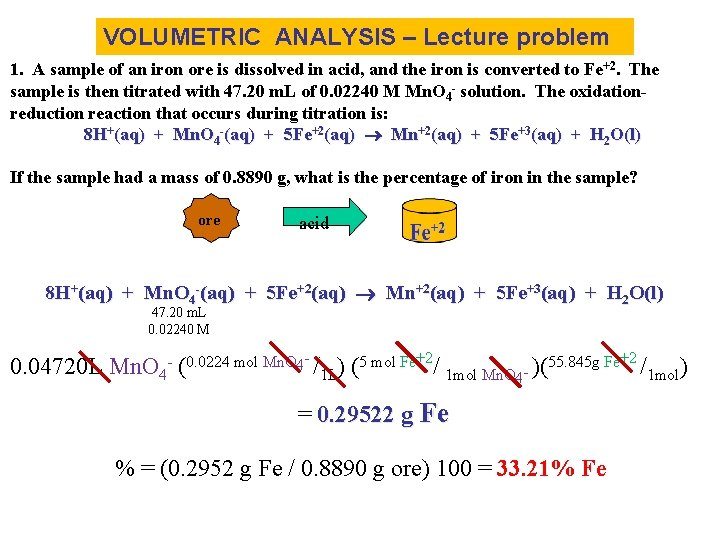VOLUMETRIC ANALYSIS – Lecture problem 1. A sample of an iron ore is dissolved in acid, and the iron is converted to Fe+2. The sample is then titrated with 47. 20 m. L of 0. 02240 M Mn. O 4 - solution. The oxidationreduction reaction that occurs during titration is: 8 H+(aq) + Mn. O 4 -(aq) + 5 Fe+2(aq) Mn+2(aq) + 5 Fe+3(aq) + H 2 O(l) If the sample had a mass of 0. 8890 g, what is the percentage of iron in the sample? ore acid 8 H+(aq) + Mn. O 4 -(aq) + 5 Fe+2(aq) Mn+2(aq) + 5 Fe+3(aq) + H 2 O(l) 47. 20 m. L 0. 02240 M 0. 04720 L Mn. O 4 - (0. 0224 mol Mn. O 4 - /1 L) (5 mol Fe+2/ 1 mol Mn. O 4 - )(55. 845 g Fe+2 /1 mol) = 0. 29522 g Fe % = (0. 2952 g Fe / 0. 8890 g ore) 100 = 33. 21% Fe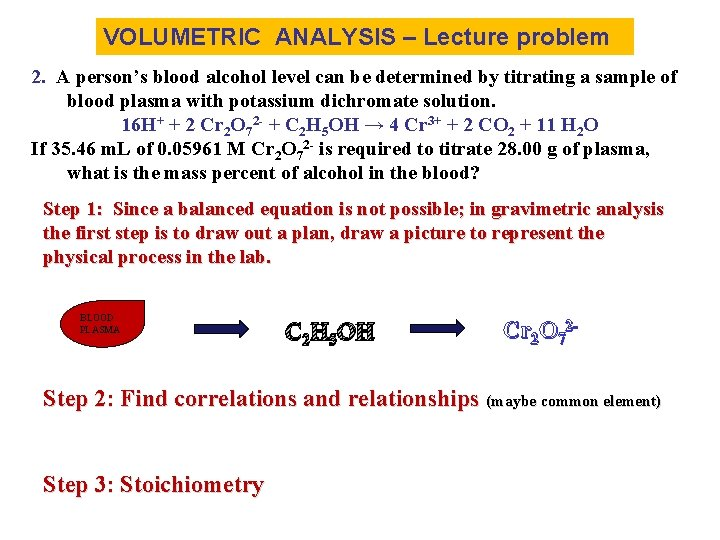VOLUMETRIC ANALYSIS – Lecture problem 2. A person’s blood alcohol level can be determined by titrating a sample of blood plasma with potassium dichromate solution. 16 H+ + 2 Cr 2 O 72 - + C 2 H 5 OH → 4 Cr 3+ + 2 CO 2 + 11 H 2 O If 35. 46 m. L of 0. 05961 M Cr 2 O 72 - is required to titrate 28. 00 g of plasma, what is the mass percent of alcohol in the blood? Step 1: Since a balanced equation is not possible; in gravimetric analysis the first step is to draw out a plan, draw a picture to represent the physical process in the lab. BLOOD PLASMA C 2 H 5 OH Cr 2 O 72 - Step 2: Find correlations and relationships (maybe common element) Step 3: Stoichiometry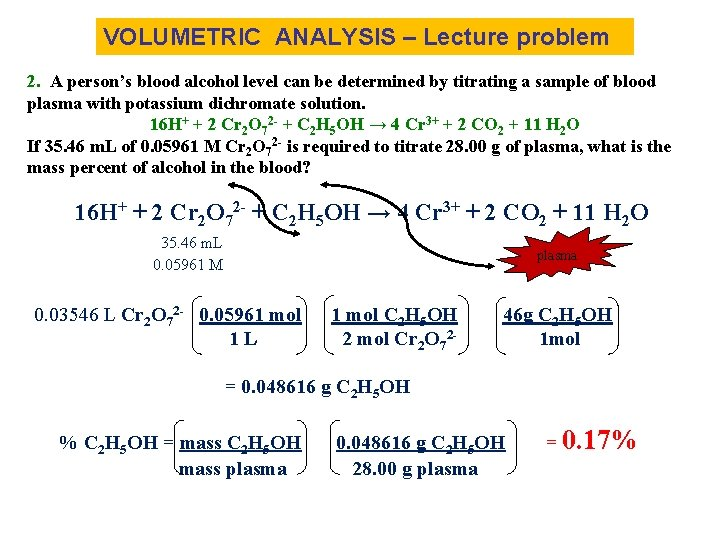VOLUMETRIC ANALYSIS – Lecture problem 2. A person’s blood alcohol level can be determined by titrating a sample of blood plasma with potassium dichromate solution. 16 H+ + 2 Cr 2 O 72 - + C 2 H 5 OH → 4 Cr 3+ + 2 CO 2 + 11 H 2 O If 35. 46 m. L of 0. 05961 M Cr 2 O 72 - is required to titrate 28. 00 g of plasma, what is the mass percent of alcohol in the blood? 16 H+ + 2 Cr 2 O 72 - + C 2 H 5 OH → 4 Cr 3+ + 2 CO 2 + 11 H 2 O 35. 46 m. L 0. 05961 M plasma 0. 03546 L Cr 2 O 72 - 0. 05961 mol 1 L 1 mol C 2 H 5 OH 2 mol Cr 2 O 72 - 46 g C 2 H 5 OH 1 mol = 0. 048616 g C 2 H 5 OH % C 2 H 5 OH = mass C 2 H 5 OH mass plasma 0. 048616 g C 2 H 5 OH 28. 00 g plasma = 0. 17%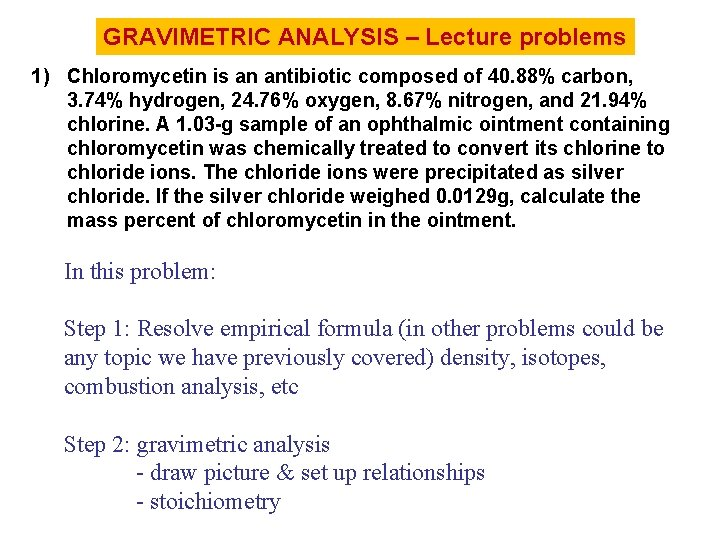GRAVIMETRIC ANALYSIS – Lecture problems 1) Chloromycetin is an antibiotic composed of 40. 88% carbon, 3. 74% hydrogen, 24. 76% oxygen, 8. 67% nitrogen, and 21. 94% chlorine. A 1. 03 -g sample of an ophthalmic ointment containing chloromycetin was chemically treated to convert its chlorine to chloride ions. The chloride ions were precipitated as silver chloride. If the silver chloride weighed 0. 0129 g, calculate the mass percent of chloromycetin in the ointment. In this problem: Step 1: Resolve empirical formula (in other problems could be any topic we have previously covered) density, isotopes, combustion analysis, etc Step 2: gravimetric analysis - draw picture & set up relationships - stoichiometry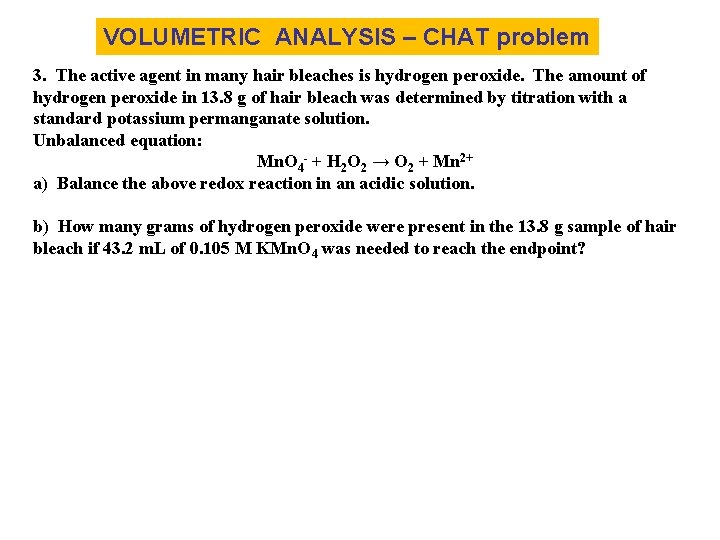VOLUMETRIC ANALYSIS – CHAT problem 3. The active agent in many hair bleaches is hydrogen peroxide. The amount of hydrogen peroxide in 13. 8 g of hair bleach was determined by titration with a standard potassium permanganate solution. Unbalanced equation: Mn. O 4 - + H 2 O 2 → O 2 + Mn 2+ a) Balance the above redox reaction in an acidic solution. b) How many grams of hydrogen peroxide were present in the 13. 8 g sample of hair bleach if 43. 2 m. L of 0. 105 M KMn. O 4 was needed to reach the endpoint?ACTIVITY on Gravimetric Analysis 1. Impure nickel can be purified by first forming the compound Ni(CO)4, which is then decomposed by heating to yield very pure nickel. Metallic nickel reacts with gaseous carbon monoxide as follows: Ni (s) + 4 CO (g) → Ni(CO)4 (g) Other metals present do not react. If 94. 2 g of a metal mixture produces 98. 4 g of Ni(CO)4, what is the mass percent of nickel in the original sample? 2. A 1. 0000 g sample of XI 2 is dissolved in water, and excess silver nitrate is added to precipitate all if the iodide as silver iodide. The mass of the dry Ag. I, is found to be 1. 375 g. Calculate the molar mass of X.